The HyperbolaCustom SearchTHE HYPERBOLA A hyperbola is a conic section with an eccentricity greater than 1. The formulas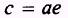And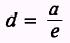developed in the section concerning the ellipse were derived so that they are true for any value of eccentricity. Thus, they are true for the hyperbola as well as for an ellipse. Since e is greater than 1 for a hyperbola, thenTherefore c > a > d.Figure 2-17.-The hyperbola. According to this analysis, if the center of symmetry of a hyperbola is the origin, then the foci lies farther from the origin than the directrices. An inspection of figure 2-17 shows that the curve never crosses the Y axis. Thus the solution for the value of b, the semiminor axis of the ellipse, yields no real value for b. In other words, b is an imaginary number. This can easily be seen from the equationsince c > a for a hyperbola. However, we can square both sides of the the above equation, and since the square of an imaginary number is a negative real number we write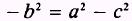or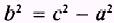and, since c = ae,Now we can use this equation to obtain the equation of a hyperbola from the following equation, which was developed in the section on the ellipse: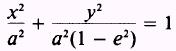and sincewe haveThis is a standard form for the equation of a hyperbola with its center, O, at the origin. The solution of this equation for y giveswhich shows that y is imaginary only when x2 < a2. The curve, therefore, lies entirely beyond the two lines x = � a and crosses the X axis at V1 (a,0) and V2( - a,0), the vertices of the hyperbola. The two straight lines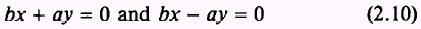can be used to illustrate an interesting property of a hyperbola. The distance from the line bx - ay = 0 to the point (x1,y1) on the curve is given by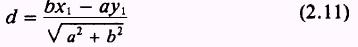Since (x1,y1) is on the curve, its coordinates satisfy the equationwhich may be written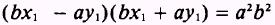orNow substituting this value into equation (2.11) gives usAs the point (x1,y1) is chosen farther and farther from the center of the hyperbola, the absolute values for x, and y, will increase and the distance, d, will approach zero. A similar result can easily be derived for the line bx + ay = 0. The lines of equation (2.10), which are usually writtenare called the asymptotes of the hyperbola. They are very important in tracing a curve and studying its properties. The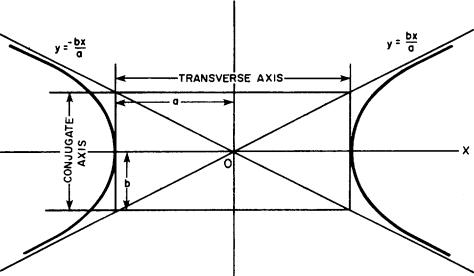Figure 2-18.-Using asymptotes to sketch a hyperbola. asymptotes of a hyperbola, figure 2-18, are the diagonals of the rectangle whose center is the center of the curve and whose sides are parallel and equal to the axes of the curve. The focal chord of a hyperbola is equal to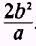. Another definition of a hyperbola is the locus of all points in a plane such that the difference of their distances from two fixed points is constant. The fixed points are the foci, and the constant difference is 2a. The nomenclature of the hyperbola is slightly different from that of an ellipse. The transverse axis is of length 2a and is the distance between the intersections (vertices) of the hyperbola with its focal axis. The conjugate axis is of length 2b and is perpendicular to the transverse axis. Whenever the foci are on the Y axis and the directrices are lines of the form y = � k, where k is a constant, the equation of the hyperbola will readThis equation represents a hyperbola with its transverse axis on the Y axis. Its asymptotes are the lines by - ax = 0 and by +ax=0 orThe properties of the hyperbola most often used in analysis of the curve are the foci, directrices, length of the focal chord, and the equations of the asymptotes. Figure 2-17 shows that the foci are given by the points F, (c,0) and FZ ( - c,0) when the equation of the hyperbola is in the form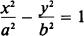If the equation were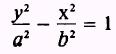the foci would be the points (0,c) and (0, -c). The value of c is either determined from the formula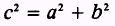or the formulaFigure 2-17 also shows that the directrices are the lines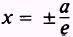or, in the case where the hyperbolas open upward and downward,. This was also given earlier in this discussion as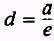.Integrated Publishing, Inc. - A (SDVOSB) Service Disabled Veteran Owned Small Business